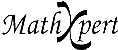# Help With Math: Purveyors of MathXpert

-->### Do I need Algebra Assistant, or Precalculus Assistant, or Calculus Assistant?

Precalculus Assistant includes algebra, and Calculus Assistant includes algebra and precalculus.

Algebra Assistant covers all of algebra, up to but not including exponential and logarithmic functions. Precalculus Assistant covers these as well as trigonometric functions and complex numbers. Calculus is about limits, derivatives, and integrals.

Each Assistant comes with problem sets on a variety of topics, but you can also type in your own problems, or a teacher can prepare a problem file. The only restrictions on what problems you can enter are that Algebra Assistant won't accept trigonometric functions such as sine and cosine, and neither Algebra Assistant nor Precalculus Assistant will accept limits, derivatives, and integrals, which require Calculus Assistant. Below is a more explicit list of topics for which the three Assistants are well suited and for which they supply problem sets.

Algebra Assistant covers all the topics usually covered in a one-year algebra course:

• linear functions and systems of linear equations
• polynomials (graphing, simplification and factoring)
• rational expressions and equations (common denominators, compound fractions, equations involving fractions)
• exponents (numerical, simple, negative, fractional)
• radical expressions (simplification, converting to and from rational exponents, solving equations)
• quadratic equations and functions (completing the square, the quadratic formula, factoring, graphing)
• inequalities (linear, polynomial, involving absolute value, involving fractions and roots)
• conic sections

Precalculus Assistant covers the following topics, in addition to those in Algebra Assistant:
• advanced algebra (sigma notation, binomial theorem, cubic equations)
• logarithms and exponential functions (change of base, simplification, solving exponential and logarithmic equations)
• complex numbers (complex arithmetic, polar form, de Moivre's theorem, complex roots, complex quadratic equations, complex trig and log functions)
• basic trigonometry (evaluating trig functions, basic identities, simplifying trigonometric expressions, solving simple trigonometric equations, addition formulas, double angle formulas, waves, graphing the trigonometric functions)
• advanced trigonometry (half-angle identities, trigonometric product and factor identities, inverse trigonometric functions, solving trigonometric equations, graphing the inverse trigonometric functions)
• hyperbolic functions (identities, graphing)
• matrices (row operations, Gauss-Jordan elimination, solving equations using the matrix inverse)
Calculus Assistant covers the following topics, in addition to those in Algebra Assistant and Precalculus Assistant.
• limits (of polynomials, trig functions, rational functions, including limits at infinity and infinite limits)
• definition of the derivative
• differentiating polynomials and trigonometric functions
• the chain rule
• higher order derivatives
• implicit differentiation
• related rate problems
• maxima and minima
• limits of exponentials and logarithms
• L'Hospital's rule
• differentiation of exponentials, logarithms, inverse trigonometric functions, hyperbolic functions
• elementary techniques of integration (substitution and integration by parts)
• advanced techniques of integration (trigonometric integrals, partial fractions, trigonometric substitutions, rationalizing substitutions)
• improper integrals
• graphical solution of differential equations